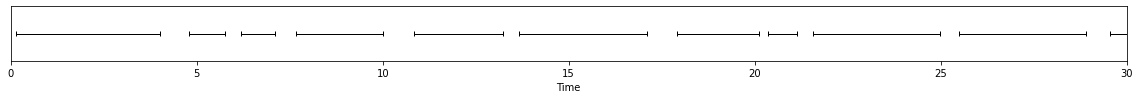# Streaming voice activity detection with pyannote.audio

Hacking with `streamz` library for real-time voice activity detection

Author

Hervé Bredin

Published

August 5, 2021

In this blog post, I describe an early attempt at performing live voice activity detection with pyannote.audio pretrained segmentation model.

### Requirements

Code
``````# setting up for pretty visualization

%matplotlib inline
import matplotlib.pyplot as plt

from pyannote.core import notebook, Segment, SlidingWindow
from pyannote.core import SlidingWindowFeature as SWF
notebook.crop = Segment(0, 10)

def visualize(features):
figsize = plt.rcParams["figure.figsize"]
plt.rcParams["figure.figsize"] = (notebook.width, 2)
notebook.plot_feature(features)``````

## Rolling audio buffer

Let us assume that the audio stream is given as a 5s rolling buffer.
Here, we are going to fake it by sliding a 5s window over the duration of an audio file.

``````from pyannote.audio.core.io import Audio, AudioFile

class RollingAudioBuffer(Audio):
"""Rolling audio buffer

Parameters
----------
sample_rate : int
Sample rate
duration : float, optional
Duration of rolling buffer. Defaults to 5s.
step : float, optional
Delay between two updates of the rolling buffer. Defaults to 1s.

Usage
-----
>>> buffer = RollingAudioBuffer()("audio.wav")
>>> current_buffer = next(buffer)
"""
def __init__(self, sample_rate=16000, duration=5.0, step=1.):
super().__init__(sample_rate=sample_rate, mono=True)
self.duration = duration
self.step = step

def __call__(self, file: AudioFile):

# duration of the whole audio file
duration = self.get_duration(file)

# slide a 5s window from the beginning to the end of the file
window = SlidingWindow(start=0., duration=self.duration, step=self.step, end=duration)
for chunk in window:
# for each position of the window, yield the corresponding audio buffer
# as a SlidingWindowFeature instance
waveform, sample_rate = self.crop(file, chunk, duration=self.duration)
resolution = SlidingWindow(start=chunk.start,
duration=1./self.sample_rate,
step=1./sample_rate)
yield SWF(waveform.T, resolution)``````

We start by initializing rolling buffer on a sample file:

``````MY_AUDIO_FILE = "DH_0001.flac"
buffer = RollingAudioBuffer()(MY_AUDIO_FILE)``````

Each subsequent call to `next(buffer)` returns the current content of the 5s rolling buffer:

``next(buffer)``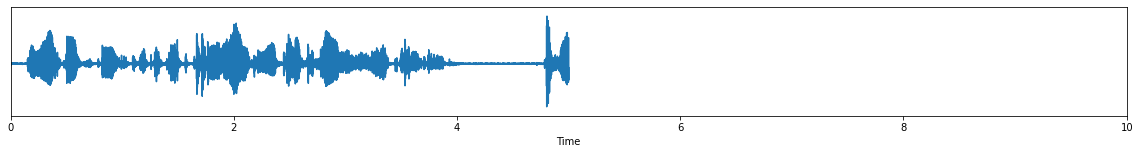``next(buffer)``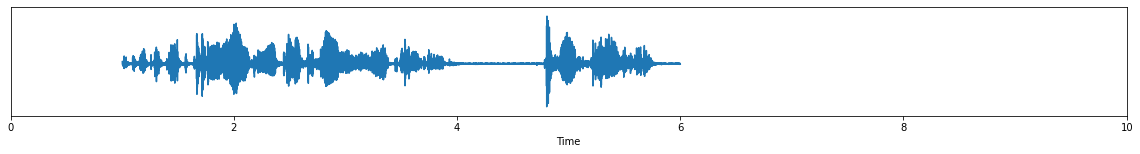``next(buffer)``For illustration purposes, we also load the manual voice activity reference.

``````from pyannote.database.util import load_rttm
reference``````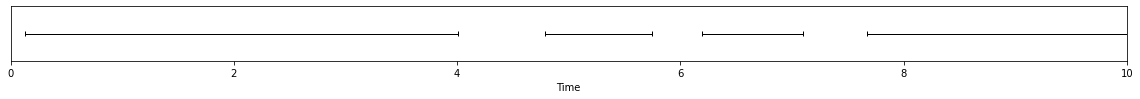## Pretrained voice activity detection model

`pyannote.audio` comes with a decent pretrained segmentation model that can be used for voice activity detection.

``````import torch
import numpy as np
from pyannote.audio import Model

class VoiceActivityDetection:

def __init__(self):
self.model = Model.from_pretrained("pyannote/segmentation")
self.model.eval()

def __call__(self, current_buffer: SWF) -> SWF:

# we start by applying the model on the current buffer
waveform = current_buffer.data.T
segmentation = self.model(waveform[np.newaxis]).numpy()

# temporal resolution of the output of the model
resolution = self.model.introspection.frames

# temporal shift to keep track of current buffer start time
resolution = SlidingWindow(start=current_buffer.sliding_window.start,
duration=resolution.duration,
step=resolution.step)

# pyannote/segmentation pretrained model actually does more than just voice activity detection
# see https://huggingface.co/pyannote/segmentation for more details.
speech_probability = np.max(segmentation, axis=-1, keepdims=True)

return SWF(speech_probability, resolution)``````
``vad = VoiceActivityDetection()``

Let us try this thing on current buffer:

``````current_buffer = next(buffer)
current_buffer````````vad(current_buffer)````reference``## Building a basic streaming pipeline with `streamz`

We now have a way to stream audio and apply voice activity detection.
According to its documentation, `streamz` seems like a good option to do that:

Streamz helps you build pipelines to manage continuous streams of data.

Let us start by creating a `Stream` that will ingest the rolling buffer and apply voice activity detection anytime the buffer is updated.

``````from streamz import Stream
source = Stream()

We re-initialize the audio buffer from the start of the file and push the rolling buffer into the pipeline:

``````buffer = RollingAudioBuffer()(MY_AUDIO_FILE)
source.emit(next(buffer))``````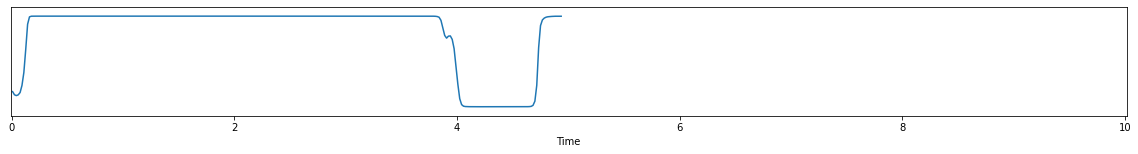``source.emit(next(buffer))``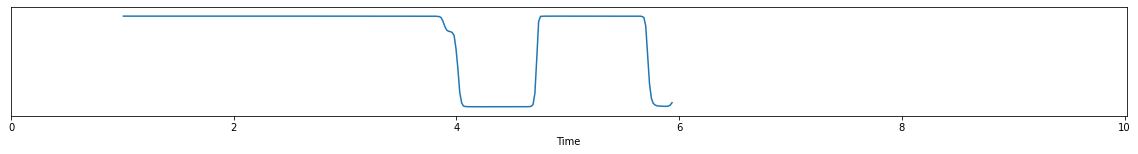``source.emit(next(buffer))````reference``## Controlling latency / accuracy trade-off

This is nice but we can do better in case the pipeline is allowed a small delay (a.k.a. latency) between when it receives the audio and when it outputs the voice activity detection scores.

For instance, if we are allowed 2s latency, we could benefit from the multiple overlapping buffers and combine them to get a better estimate of the speech probability in regions where the model is not quite confident (e.g. just before t=4s).

This is what the `Aggregation` class does.

``````from typing import Tuple, List

class Aggregation:
"""Aggregate multiple overlapping buffers with a

Parameters
----------
latency : float, optional
Allowed latency, in seconds. Defaults to 0.
"""

def __init__(self, latency=0.0):
self.latency = latency

def __call__(self, internal_state, current_buffer: SWF) -> Tuple[Tuple[float, List[SWF]], SWF]:
"""Ingest new buffer and return aggregated output with delay

Parameters
----------
internal_state : (internal_time, past_buffers) tuple
`internal_time` is a float such that previous call emitted aggregated scores up
to time `delayed_time`.  `past_buffers` is a rolling list of past buffers that
we are going to aggregate.
current_buffer : SlidingWindowFeature
New incoming score buffer.
"""

if internal_state is None:
internal_state = (0.0, list())

# previous call led to the emission of aggregated scores up to time `delayed_time`
# `past_buffers` is a rolling list of past buffers that we are going to aggregate
delayed_time, past_buffers = internal_state

# real time is the current end time of the audio buffer
# (here, estimated from the end time of the VAD buffer)
real_time = current_buffer.extent.end

# because we are only allowed `self.latency` seconds of latency, this call should
# return aggregated scores for [delayed_time, real_time - latency] time range.
required = Segment(delayed_time, real_time - self.latency)

# to compute more robust scores, we will combine all buffers that have a non-empty
# temporal intersection with required time range. we can get rid of the others as they
# will no longer be needed as they are too far away in the past.
past_buffers = [buffer for buffer in past_buffers if buffer.extent.end > required.start] + [current_buffer]

# we aggregate all past buffers (but only on the 'required' region of interest)
intersection = np.stack([buffer.crop(required, fixed=required.duration) for buffer in past_buffers])
aggregation = np.mean(intersection, axis=0)

# ... and wrap it into a self-contained SlidingWindowFeature (SWF) instance
resolution = current_buffer.sliding_window
resolution = SlidingWindow(start=required.start, duration=resolution.duration, step=resolution.step)
output = SWF(aggregation, resolution)

# we update the internal state
delayed_time = real_time - self.latency
internal_state = (delayed_time, past_buffers)

# ... and return the whole thing for next call to know where we are
return internal_state, output``````

Let’s add this new accumulator into the streaming pipeline, with a 2s latency:

``````source = Stream()
source \
.accumulate(Aggregation(latency=2.), returns_state=True, start=None) \
.sink(visualize)

buffer = RollingAudioBuffer()(MY_AUDIO_FILE)``````
``````# first [0s, 5s] buffer
current_buffer = next(buffer); current_buffer````````````# ... and its corresponding output up to 3s (= 5s - latency)
source.emit(current_buffer)``````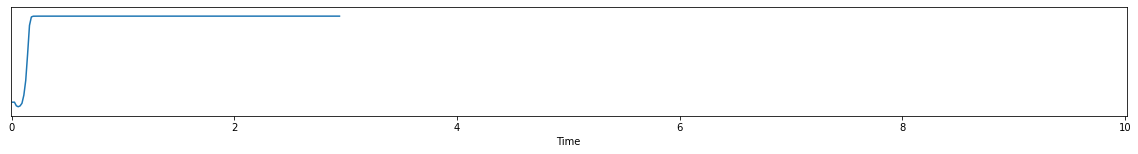``````# next [1s, 6s] buffer
current_buffer = next(buffer); current_buffer````````````# ... and its corresponding [3s, 6s - latency = 4s] output
source.emit(current_buffer)````````````# next [2s, 7s] buffer
current_buffer = next(buffer); current_buffer````````````# ... and its corresponding [4s, 7s - latency = 5s] output
source.emit(current_buffer)``````Look how the aggregation process actually refined the speech probability just before t=4s. This has been enabled by the longer latency.

## That’s all folks!

For technical questions and bug reports, please check pyannote.audio Github repository.

## Bonus: concatenating output

For visualization purposes, you might want to add an accumulator to the pipeline that takes care of concatenating the output of each step…

``````class Concatenation:

def __call__(self, concatenation: SWF, current_buffer: SWF) -> Tuple[SWF, SWF]:

if concatenation is None:
return current_buffer, current_buffer

resolution = concatenation.sliding_window
current_start_frame = resolution.closest_frame(current_buffer.extent.start)
current_end_frame = current_start_frame + len(current_buffer)

concatenation.data = np.pad(concatenation.data, ((0, current_end_frame - len(concatenation.data)), (0, 0)))
concatenation.data[current_start_frame: current_end_frame] = current_buffer.data

return concatenation, concatenation``````
``````source = Stream()
source \
.accumulate(Aggregation(latency=2.), returns_state=True, start=None) \
.accumulate(Concatenation(), returns_state=True, start=None) \
.sink(visualize)

buffer = RollingAudioBuffer()(MY_AUDIO_FILE)``````
``````notebook.crop = Segment(0, 30)
for _ in range(30):
source.emit(next(buffer))``````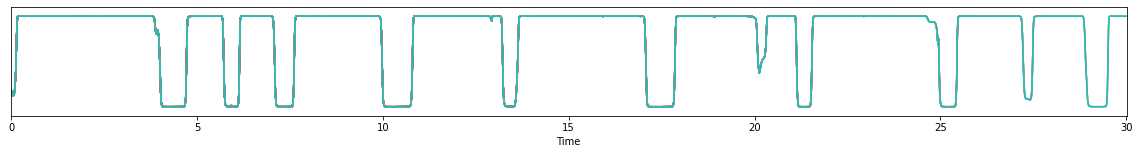``reference``